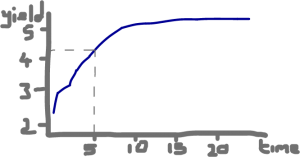A vanilla interest rate swap is an agreement to swap a fixed rate coupons made on a large notional amount against floating rate coupons on the same notional. The floating rate used may be LIBOR, EURIBOR, EONIA, etc. At the conclusion of the agreement, the notional amount of the trade is not exchanged between the parties.

Our example will be a five year swap where payments are made every 6 months using LIBOR for the floating leg and the fixed rate will be 3%. On the trade date the LIBOR rate will be recorded. This will dictate the floating payment payment to be made at the first payment date in 6 months time. At that time, the corresponding fixed payment will be 0.03 * notional_amount. For the other 9 payments, the fixed leg will continue to pay the same sum: 0.03 * notional_amount. For the floating leg, the rate to be paid will continue to be the LIBOR rate snapped at the previous payment date i.e.

```Payment    Time (months)    Rate (as at month)
1                6                     0
2               12                     6
3               18                    12

...              ...                   ...

```

The price of each leg is as follows:

PV = ce- 0.5 R1 + ce- 1.0 R2

Where c (for coupon) is the notional amount

So the value of the fixed leg is a straight forward calculation. The value of the floating leg is the same but uses rates taken from the swap curve which we need to build.

## Building a swap curve

When valuing the floating leg on the trade date, we need to take rates from the swap curve.This is built from a variety of products taken from exchanges where the products are of similar credit worthiness.

The rates that make the curve are "n-year zero rates", often called zero rates. A zero rate is the rate an investment (like an IR bond) would pay when all of the interest was paid at maturity with the repayment of a principal amount. So we can see a 5 year investment should return at a rate of about 4.2% from our curve above. Where do these rates to make the curve come from:

#### Short-end

These are the easy points of the swap curve. In the near future, there are many publicly quoted bonds that are nearing expiry where there are no remaining coupons to be paid. It can therefore be assumed that the rates offered are purely zero-rates. They just need to be converted in to continually compounding interest.

A bond with a principal of \$100, maturing in 3 months (0.25 years) has no more coupons to pay and in the market today is priced at \$97.50. Therefore it will return \$2.5 over the 3 months and so its quarterly compounding interest is

(4 x 2.5 / 97.5 = 0.10256 or 10.256% per annum

Expressed in continually compounding interest, this is

R3m = 4 ln (1 + (0.10256 / 4)) = 0.10127 or  10.127%

#### Long-end

Further out in the future, bonds will have coupons still to be paid midway through their lifecycle. These can make points on the swap curve but first have to be converted to a zero-rate IR products: adjusted to be a single bullet payment at maturity with the correct equivalent interest rate. This new interest rate is called the "implied zero-coupon rate" or just "zero rate". Bonds are added to the curve one after another from the earlier maturing bonds to the last maturing, going out 30 - 50 years from now. To take an example:

We have a bond expiring in 6 months (0.5 years). It pays 4 coupons annually of \$8 (\$2 each time) with a \$100 principal. Today it is available on the market at \$96.00. Therefore the payments on this bond will be:

3 months: \$2
6 months: \$102

So for the zero rate at 6 months, this equation allows us to infer the 6m continuously compounding rate:

2 e0.10127 x 0.25 + 2 e0.5 R = 96

∴  R6m = 2ln(96 - 2 e0.10127 x 0.25 / 2)